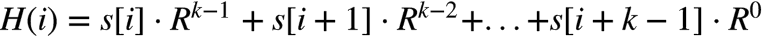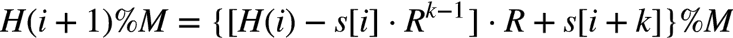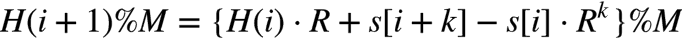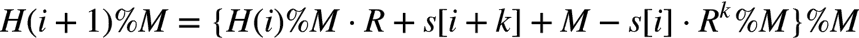1. Hash函数

正整数

M一般是素数，否则可能无法利用key中包含的所有信息。如key是十进制数而M是10的k次方，那么只能利用key的后K位，不均匀，增加碰撞概率。

字符串

%也适用于字符串，只需要将字符串看成一个若干位R进制的整数，不同的是R并不一定非得大于字符集的个数，可以适当进行调整，常常选取质数。

public int hash(String s,int M){
int hash = 0;
int R = 101;    //进制
/*
Horner's rule:
hash(abc)=a*R^2 + b*R^1 + c*R^0 = ((a*R + b) * R) + c

*/
for(int i=0;i<s.length();i++){
hash = R * hash + s.charAt(i);
}
return hash % M;
}

hash = (R * hash + s.charAt(i)) % M;

(a+b)%n = (a%n + b%n) %n;
(a-b)%n = (a%n - b%n + n) %n;
(a*b)%n = (a%n * b%n) %n;

2. Java的hashCode()

JDK为String类的hashCode提供了默认的实现：

/**
* Returns a hash code for this string. The hash code for a
* <code>String</code> object is computed as
* <blockquote><pre>
* s*31^(n-1) + s*31^(n-2) + ... + s[n-1]
* </pre></blockquote>
* using <code>int</code> arithmetic, where <code>s[i]</code> is the
* <i>i</i>th character of the string, <code>n</code> is the length of
* the string, and <code>^</code> indicates exponentiation.
* (The hash value of the empty string is zero.)
*
* @return  a hash code value for this object.
*/
public int hashCode() {
int h = hash;
if (h == 0) {
int off = offset;
char val[] = value;
int len = count;

for (int i = 0; i < len; i++) {
h = 31*h + val[off++];
}
hash = h;
}
return h;
}

int hash(Object x){
return (x.hashCode() & 0x7fffffff) % M;
}

3. 碰撞

1. 元素较少且比较随机时，使用一个巨大的映射空间（比如M=一个巨大的素数、直接MD5等等，空间不需保存不占内存），碰撞概率为1/M

碰撞的概率很低很低，相信概率的力量。

2. 元素很多时，进行k次hash，降低碰撞率

BloomFilter就是这么干的，加入的元素个数在某个阈值内时能保证错误率在可接受范围内，再多的话碰撞的概率就不行了。

4. Rabin-Karp子字符串查找算法

Rabin-Karp算法的关键思想是 某一子串的hash值可以根据上一子串的hash在常数时间内计算出来，这样比对的时间复杂度可以降为O(n-k)。Rabin-Karp对字符串的hash算法和上面描述的一样（按整数进制解析再求模），假设原字符串为s，H(i)表示第i个字符开始的k个子字符串的hash值，即，（先不考虑%M），则，时间为常数。M 一般选取一个非常大的数字，子串的数目相对而言非常少，产生散列碰撞的概率为 1/M，可以忽略不计。

package algorithm.string;

import java.math.BigInteger;

public class RabinKarp {

private static long M = 1000000000000000003L;   //集合空间大小，一个很大的素数
private static int R = 31;  //进制
{
//M = BigInteger.valueOf((long) Math.pow(10, 18)).nextProbablePrime().longValue();
}

private long patternHash;
private long RK; // R^K % M，用于减去第一个数时的计算
private int K; // 模式字符串的长度

public RabinKarp(String pattern) {
patternHash = hash(pattern);
K = pattern.length();

// 计算RM
RK = 1;
for (int i = 0; i < K; i++) {
RK = (R * RK) % M;
}
}

public int search(String txt) {
long substrHash = hash(txt, 0, K);
if (substrHash == patternHash) return 0;    //一开始就匹配成功
for (int i = 1; i + K <= txt.length(); i++) {
// H(i+1) % M = [H(i) % M * R + s[i+k] - s[i] * R^k % M + M] % M
substrHash = (substrHash * R  + txt.charAt(i + K - 1) - txt.charAt(i - 1) * RK % M  + M ) % M;
if (substrHash == patternHash)
return i;
}
return -1;
}

// Horner rule 计算字符串hash值
private long hash(String str, int start, int length) {
long hash = 0;
for (int i = start; i < length; i++) {
hash = (hash * R + str.charAt(i)) % M;
}
return hash;
}

private long hash(String str) {
return hash(str, 0, str.length());
}

public static void main(String[] args) {
String pattern = "y similar t";
RabinKarp rk = new RabinKarp(pattern);
String txt = "Technically, this algorithm is only similar to the true number in a non-decimal";
System.out.println(txt);
int index = rk.search(txt);
if (index >= 0) {
for (int i = 0; i < index; i++) {
System.out.print(" ");
}
System.out.print(pattern);
}

}
}

Technically, this algorithm is only similar to the true number in a non-decimal
y similar t

5. 二维扩展

Rabin-Karp 算法可以扩展到二维，可用于二维数组、图像的查找。基本思想如下：